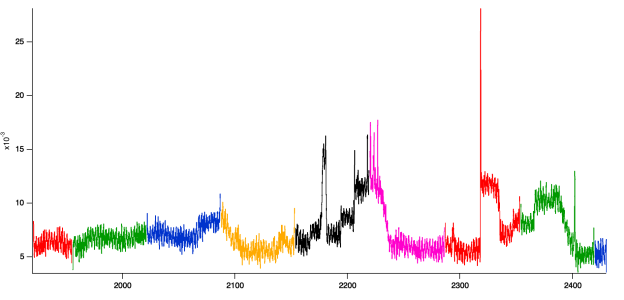# Remapping some wavesHi,

Here is the problem I have a set of waves which are measurements that have been broken up and I want to reconstruct a single wave.  The waves have different  x scaling and there is a break between them were no data was collected.  There are also much higher frequency than I actually need so I can down sample.

I have created a master wave that has x scaling that represents the range of the samples. I found out that I cannot use straight forward x mapping within functions and am forced to use point scaling. It compiles ok, but when I run it I keep getting out of range errors on the last assignment.

Function MaptoSingle(sensor)
wave sensor

String runs =nameofwave(sensor)
sensor = nan
setdataFolder \$("root:"+runs)
String listOfRuns
listOfRuns = wavelist("*_sm",";","")
variable index,maxindex,startpoint,endpoint,endx
maxindex = itemsInList(listOfRuns)
for(index=0;index<maxindex;index+=1)
wave subset = \$(stringfromList(index,listOfRuns))
startpoint =x2pnt(sensor,leftx(subset))
//x value at the end of the subset wave
endx=pnt2x(subset,(numpnts(subset)-1))
endpoint = x2pnt(sensor,endx)
sensor[startpoint,endpoint] = subset(pnt2x(sensor,p))
endfor
setdatafolder root:
end

I am trying to figure where things go astray.

Pointers or a better way to do this would be most appreciated.

AndyIt's hard to debug without having the data, but one way you might be getting that out of range error is if the wave "sensor" doesn't include the full range of x values present all of the "subset" waves. You could assure that "sensor" will have a big enough range by adding something like the following to your code:

Function MaptoSingle(sensor,dt)
wave sensor;
//new, specify the sampling rate you want:
Variable dt //final x scaling for sensor wave

String runs =nameofwave(sensor)
sensor = nan
setdataFolder \$("root:"+runs)
String listOfRuns
listOfRuns = wavelist("*_sm",";","")
variable index,maxindex,startpoint,endpoint,endx
maxindex = itemsInList(listOfRuns)

//new: pre-check for minX and maxX needed
Variable minX=inf,maxX=-inf
for(index=0;index<maxindex;index+=1)
wave subset = \$(stringfromList(index,listOfRuns))
minX = min(minX,dimDelta(subset,0))
maxX = max(maxX,pnt2x(subset,dimsize(subset,0)-1))
endfor

//new: resize the output wave as needed
Variable outPnts = 1+(maxX-minX)/dt
redimension/n=(outPnts) sensor  //using redimension to maintain the type of sensor, would be better still to use the wave type of one of the input waves
sensor = nan
setscale/p x,minX,dt,waveunits(subset,0),sensor //uses a source wave's unitStr in case it has one

//(unchanged from here)
for(index=0;index<maxindex;index+=1)
wave subset = \$(stringfromList(index,listOfRuns))
startpoint =x2pnt(sensor,leftx(subset))
//x value at the end of the subset wave
endx=pnt2x(subset,(numpnts(subset)-1))
endpoint = x2pnt(sensor,endx)
sensor[startpoint,endpoint] = subset(pnt2x(sensor,p))
endfor
setdatafolder root:
end

Hi,

Let me study your code a bit more.  Since the scaling is slightly different with each input wave, I need to keep it constant.  I have set up the receiving wave to have excess span so that is not the issue (I think).  I think the problem is in the pnt2x and x2pnt operations giving rounding errors that can take things out range.  I have added another variable to get a full sense of the span since the p assignment does let me see into the calculations. The real range of the subset wave is 1921 to 1955, notice the conversion process has now set the final endpoint when converted back to the original x scaling at 1955.00256..  This is out the range and causing the failure (I think). Now I have think about how to trap at both ends.

Hi,

Made some modifications that trapped for the beginning and ending points of my receiver wave range.  I use a combination of min and max statements to make the range is always within the range of the subset wave.  This works but was way more painful then it needed to be.  I had thought the use of wave scaling would be a benefit, but with great power comes great bugs.

Function MaptoSingle(sensor)
wave sensor

String runs =nameofwave(sensor)
sensor = nan
setdataFolder \$("root:"+runs)
String listOfRuns
listOfRuns = wavelist("*_sm",";","")
variable index,maxindex,startpoint,endpoint,subStart,Subend
maxindex = itemsInList(listOfRuns)
for(index=0;index<maxindex;index+=1)
wave subset = \$(stringfromList(index,listOfRuns))
substart = leftx(subset)
startpoint =x2pnt(sensor,leftx(subset))
//x value at the end of the subset wave
endpoint = x2pnt(sensor,endx(subset))
subend = endx(subset)
sensor[startpoint,endpoint] = subset(min(max(pnt2x(sensor,p),substart),subend))
endfor
setdatafolder root:
end

function endx(wavein)
wave wavein

return pnt2x(wavein,(numpnts(wavein)-1))
end

Andy

Would ScaletoIndex and IndextoScale be of any clearer benefit somewhere?

Alternatively, can you ...

* create an (free) x-wave for all subsets that contains the x-values at the points: duplicate/O subset, xwaveic; xwaveic = x

* concatenate the subsets to sensor and the xwaveic to xwavefull

* use an alogrithm to "fill in the blanks" in the xwavefull (i.e. add points to keep consistent increments) and correspondingly add "blanks" in the sensor wave at those locations

* map the x-wave as the x-scaling for the sensor wave

Or alternatively,

I can ask the client to do a better job taking the data.  The sensor is a Keyence laser sensor and it goes into their control box which has limit of 100,000 data points.  The sample is moving underneath and they opted to keep the sample moving while the saved cleared the buffer to avoid stop start issues.  I have suggested that they stream the data to a real data collection system and this would not be a problem.

Andy

To butcher William Shakespeare:  "The fault does not lie in our stars but in ourselves (clients)."  They  tend to be penny wise and pound foolish. In this particular instance it may strain IP to record data directly and I have done this often. There are 4 sensors and the data comes every 40ms. In general my projects are very ad hoc and I find it much easier to do everything possible from within IP so I don't need to be an expert in too many systems and have built many a metrology tool with IP as the controlling software.

Andy